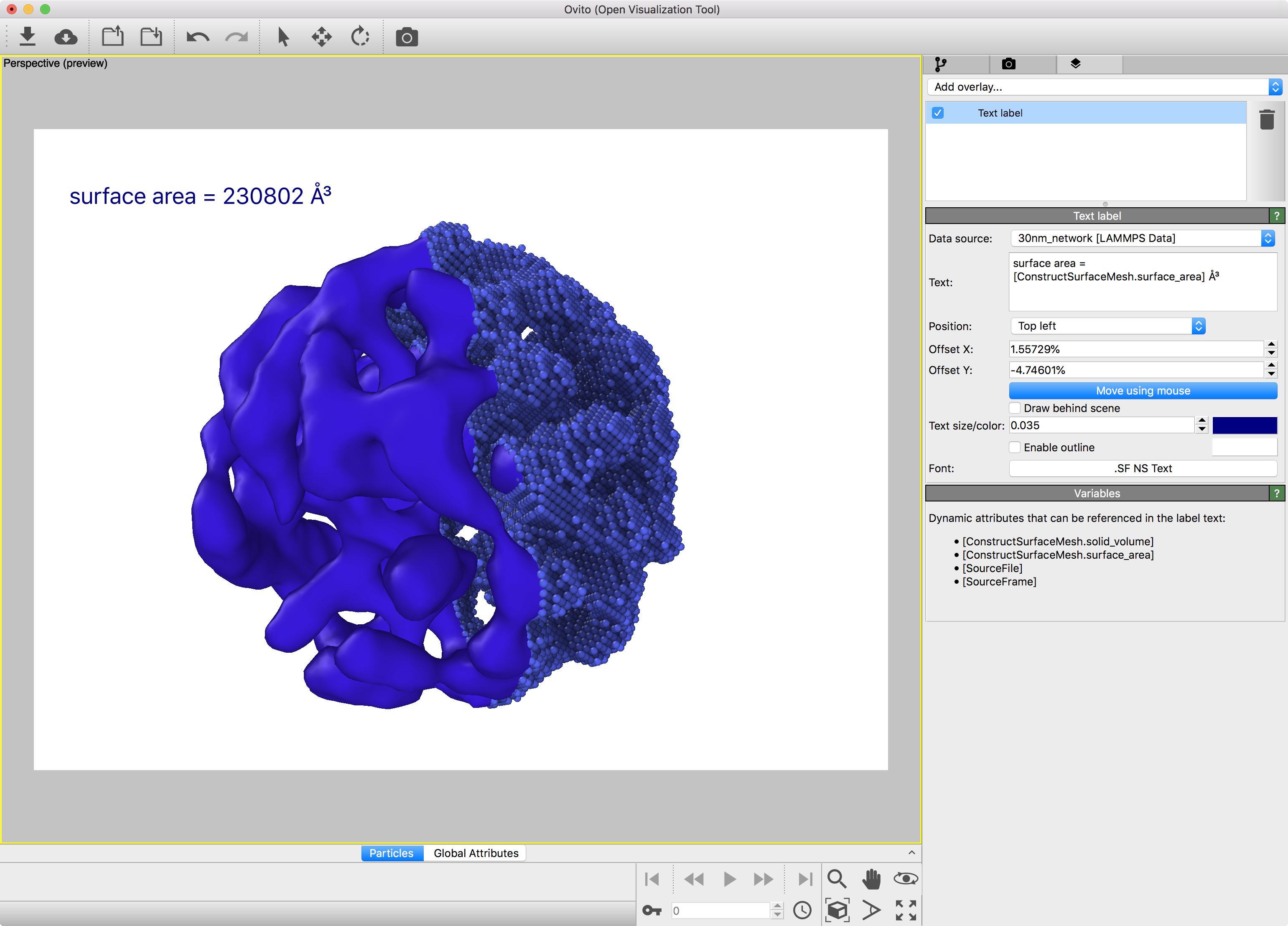### Spatial binning modifier

OVITO allows you to ….

In the first three cases the modifier generates a one-dimensional grid with bins aligned perpendicular to the selected simulation cell vector. In the last three cases the modifier generates a two-dimensional grid with bins aligned perpendicular to both selected simulation cell vectors (i.e. parallel to the third vector).### How can I do this?

• Download and import the example file …. From the four viewport windows now choose the Perspective view and enlarge it by clicking on the Maximize Active Viewport button.
•

`from ovito.data import *`
`import numpy as np`
`import matplotlib.pyplot as plt`
`import matplotlib`
`def modify(frame, data):`
`    grid = data.grids['binning[Potential Energy]']`
`    array = grid['Potential Energy'] `
`    array_2d = np.reshape(array, (grid.shape, grid.shape) )`

`    plt.figure()`
`    matplotlib.rcParams.update({'font.size': 18})`
`    im = plt.imshow(array_2d, cmap='jet', aspect='equal', origin='lower')`
`    plt.xlabel('coordinate 1')`
`    plt.ylabel('coordinate 2')`
`    plt.colorbar(shrink = 0.65)`
`    plt.savefig('{}{}.pdf'.format(grid.title, frame), bbox='tight')`